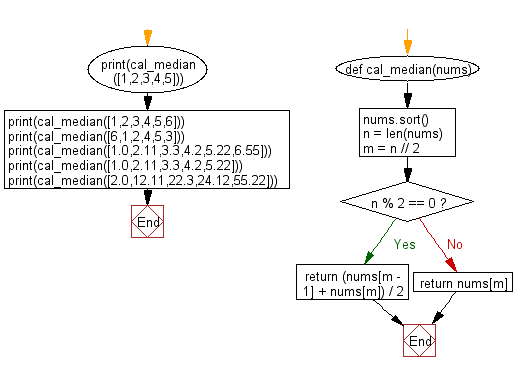﻿ Python: Calculate the median from a list of numbers - w3resource# Python: Calculate the median from a list of numbers

## Python Basic - 1: Exercise-82 with Solution

Write a Python program to calculate the median from a list of numbers.

From Investopedia:
What is the Median?
The median is the middle number in a sorted, ascending or descending, list of numbers and can be more descriptive of that data set than the average.

KEY TAKEAWAYS
• The median is the middle number in a sorted, ascending or descending, list of numbers and can be more descriptive of that data set than the average.
• The median is sometimes used as opposed to the mean when there are outliers in the sequence that might skew the average of the values.
• If there is an odd amount of numbers, the median value is the number that is in the middle, with the same amount of numbers below and above.
• If there is an even amount of numbers in the list, the middle pair must be determined, added together, and divided by two to find the median value.

Median Example:

To find the median value in a list with an odd amount of numbers, one would find the number that is in the middle with an equal amount of numbers on either side of the median. To find the median, first arrange the numbers in order, usually from lowest to highest.
For example, in a data set of {3, 13, 2, 34, 11, 26, 47}, the sorted order becomes {2, 3, 11, 13, 26, 34, 47}. The median is the number in the middle {2, 3, 11, 13, 26, 34, 47}, which in this instance is 13 since there are three numbers on either side.
To find the median value in a list with an even amount of numbers, one must determine the middle pair, add them, and divide by two. Again, arrange the numbers in order from lowest to highest.
For example, in a data set of {3, 13, 2, 34, 11, 17, 27, 47}, the sorted order becomes {2, 3, 11, 13, 17, 27, 34, 47}. The median is the average of the two numbers in the middle {2, 3, 11, 13, 17, 26 34, 47}, which in this case is fifteen {(13 + 17) ÷ 2 = 15}.

Sample Solution:

Python Code:

``````def cal_median(nums):
nums.sort()
n = len(nums)
m = n // 2
if n % 2 == 0:
return (nums[m - 1] + nums[m]) / 2
else:
return nums[m]
print(cal_median([1,2,3,4,5]))
print(cal_median([1,2,3,4,5,6]))
print(cal_median([6,1,2,4,5,3]))
print(cal_median([1.0,2.11,3.3,4.2,5.22,6.55]))
print(cal_median([1.0,2.11,3.3,4.2,5.22]))
print(cal_median([2.0,12.11,22.3,24.12,55.22]))
``````

Sample Output:

```3
3.5
3.5
3.75
3.3
22.3
```

Pictorial Presentation:Flowchart:Python Code Editor:

Have another way to solve this solution? Contribute your code (and comments) through Disqus.

What is the difficulty level of this exercise?

Test your Programming skills with w3resource's quiz.

﻿

## Python: Tips of the Day

What is the difference between Python's list methods append and extend?

append: Appends object at the end.

```x = [1, 2, 3]
x.append([4, 5])
print (x)
```

Output:

```[1, 2, 3, [4, 5]]
```

extend: Extends list by appending elements from the iterable.

```x = [1, 2, 3]
x.extend([4, 5])
print (x)
```

Output:

```[1, 2, 3, 4, 5]
```

Ref: https://bit.ly/2AZ6ZFq